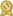# Mr. Kevin D.

## specializes in Effective tutor in Algebra, Calculus, Linear algebra,DEQs and any Math · male

"I have been tutoring students in Mathematics since I was a college student. I love working one-on-one with students and help them truly understand complex mathematical concepts and problem solving strategies. I love to see the aha! moments in students when they finally understand math. And I love to help students apply the math theory they learn to solve problems in a variety of setting. I love to help students succeed in subjects such as Calculus, Differential Equations, Linear Algebra, Algebra I, Algebra II, Geometry, or any kind of math that you need help with or are interested."

# Details

 fee: \$60 (for 60 min) travel distance: 10 miles meeting type: either in-person or online

# Contact

 website: on file

# Education and Qualifications

 certified:BYU, Math

BYU, Masters

# Subjects Tutored

Algebra 1 - Algebra 2 - Calculus - Geometry - Precalculus - Trigonometry - Statistics - SAT Math - GRE - GED - Probability - ACT Math - Differential Equations - Linear Algebra - Finite Math